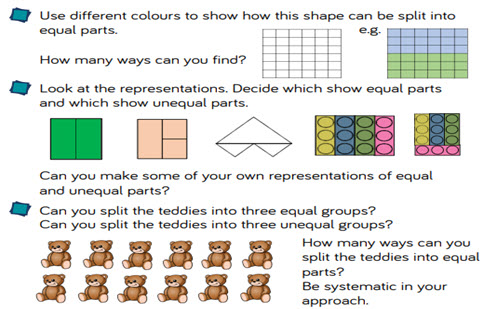# Fractions: halves, quarters, thirds and equivalence

This guide provides a sequence of small steps for exploring fractions, from recognising and finding halves, quarters and thirds through to exploring equivalence and counting in fractions.

Year level(s) Year 3, Year 4
Audience Teacher
Purpose Teaching resource, Teaching strategies
Teaching strategies and pedagogical approaches Questioning, Concrete Representational Abstract model, Explicit teaching
Keywords fractions, unit fractions, non-unit fractions, equivalence, explicit teaching

## Curriculum alignment

Curriculum connections Numeracy
Strand and focus Number, Build understanding
Topics Fractions, Multiplication and division
AC: Mathematics (V9.0) content descriptions
AC9M3N02

Recognise and represent unit fractions including 12, 13, 14, 15 and 110 and their multiples in different ways; combine fractions with the same denominator to complete the whole

AC9M4N03
Find equivalent representations of fractions using related denominators and make connections between fractions and decimal notation

AC9M4N04
Count by fractions including mixed numerals; locate and represent these fractions as numbers on number lines

Numeracy progression Interpreting fractions (P5)
Counting processes (P8)